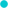# Overweight Underweight Fitness Calculator#BMI Calculator

An online body mass index calculation

feet  inches
poundsConvert Pounds to KG / KG to Pounds## How Overweight Underweight Fitness(BMI) calculator works ?

Overweight Underweight Fitness Calculator: This is used as an estimate of overweight and underweight.It is calculated the same way for men and women.
General classifications:

• Underweight: less than 18.5
• Normal weight: 18.5-24.9
• Overweight: 25-29.9
• Obesity: BMI of 30 or greater

##Age Calculators ►

Horse Age Calculator , Cow Age Calculator , Cat Age Calculator , Dog Age Calculator, Human Age Calculator 100 Days Old Calculator Date Difference Calculator